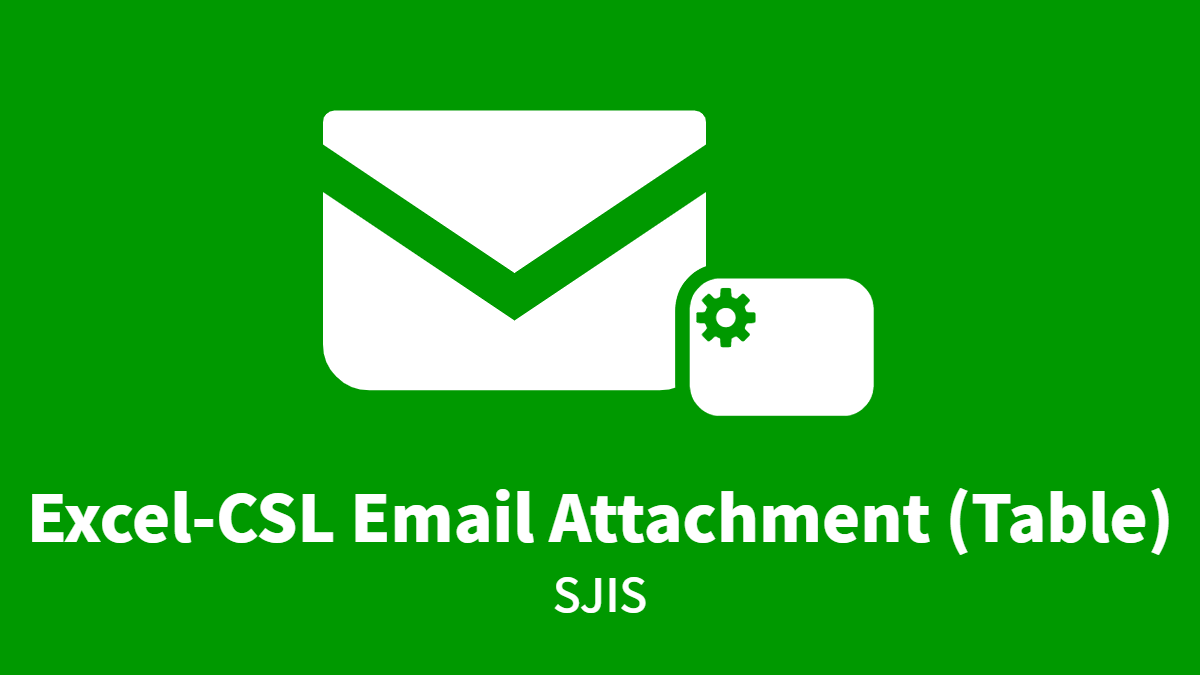Email a Excel-CSV (Table) SJIS
Converting the values in a Table type data item to an Excel CSV file (TSV), and sends it as email attachment.
Configs
• A: Select TABLE DATA *
• B: Set THROW COL IDs (e.g. “0,1,5,3,6”) *
• C: Select STRING DATA for To Address *
• D: Select STRING DATA for To Name *
• E: Select STRING DATA for Email Subject *
• G: Set From Name (e.g. “Accounting News”) *
• J: Select STRING DATA for Email Body *
• K: Select STRING DATA for Attachment File Name *
Script
``````//// == Config Retrieving ==
var dataIdA = configs.get( "conf_DataIdA" ); // (returns Number)
var colIds = configs.get( "conf_ColIds" ) + "";
var dataIdC = configs.get( "conf_DataIdC" ); // (returns Number)
var dataIdD = configs.get( "conf_DataIdD" ); // (returns Number)
var dataIdE = configs.get( "conf_DataIdE" ); // (returns Number)
var fromName = configs.get( "conf_FromName" );
var dataIdJ = configs.get( "conf_DataIdJ" ); // (returns Number)
var dataIdK = configs.get( "conf_DataIdK" ); // (returns Number)

//// == Data Retrieving ==
var myTable = data.get( dataIdA );
// myTable: com.questetra.bpms.core.model.formdata.ListArray
var toAddress = data.get( dataIdC ) + "";
var toName = data.get( dataIdD ) + "";
var subject = data.get( dataIdE ) + "";
var myBody = data.get( dataIdJ ) + "";
var myFileName = data.get( dataIdK ) + "";

//// == Calculating ==
if( myTable !== null){
var colIdArray = colIds.split(",");
var numOfCols = colIdArray.length;
var numOfRows = myTable.size() - 0; // 行（Tableの高さ）

var myTsv = "";
for( var i = 0; i < numOfRows; i++ ){
for( var j = 0; j < numOfCols; j++ ){
if( j != numOfCols - 1 ){
myTsv += myTable.get(i, parseInt( colIdArray[j] ) ) + "\t";
}else{
myTsv += myTable.get(i, parseInt( colIdArray[j] ) ) + "\n";
}
}
}

myTsv = myTsv.replace( /,/g, "" );
myTsv = myTsv.replace( /\t/g, "," );

emailService.begin()
.setSubject( subject )
.setBody( myBody )
.send();
}``````

• Email-SendExcelCsvSjis.xml
• Since Rhino (deprecated) is specified as the script engine, a setting error will occur even if you install this in a workflow App
• To use this Add-on, you need to change the script engine and modify the script accordingly
• A modified version is in preparation

### Notes

• “0,1,5,3,6” means changing the column order into “ID:0, ID:1, ID:5,ID:3, ID:6” before generating the CSV.
• The SJIS version consists of comma-separated-values. Although the availability of smartphone display and data import increases, comma information misses.

### 1 thought on “Email a Excel-CSV (Table) SJIS”

1. Pingback: Help: Table-Type – Questetra Support